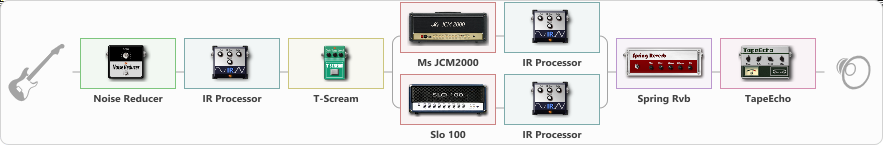# charvell

Discussion in 'ToneLib-GFX presets' started by carlinos3, Sep 16, 2023.

1. charvel
Nombre preestablecido: Plomo de metal

Comentarios:Efecto: " Reductor de ruido " ( Dinámica/Filtro ), activo - "sí"
{

"Sentidos" = 49
"Mode" = Hard
}

Effect: "IR Processor" (Cabinets), active - "yes"
{

"IR" = pafpro_dc
"Low Cut (Hz)" = 0
"Hi Cut (kHz)" = 20.0
"Mix" = 80
"Level (dB)" = 9
}

Effect: "T-Scream" (Overdrive / Distortion), active - "yes"
{

"Drive" = 22
"Tone" = 80
"Level" = 79
}

Effect: "Splitter" (Dynamics / Filter)
{

"A-Bypass" = Off
"A-Pan" = -100
"A-Level" = 55
"B-Bypass" = Off
"B-Pan" = 100
"B-Level" = 55
"Width" = 0
'A' branch:
{

Effect: "Ms JCM2000" (Amp simulators), active - "yes"
{

"Gain" = 100
"Bass" = 50
"Middle" = 50
"Treble" = 50
"Presence" = 76
"Master" = 100
"Level (dB)" = 0
}
Effect: "IR Processor" (Cabinets), active - "yes"
{

"IR" = dic-1_dc
"Low Cut (Hz)" = 0
"Hi Cut (kHz)" = 20.0
"Mix" = 100
"Level (dB)" = 2
}
}
'B' branch:
{

Effect: "Slo 100" (Amp simulators), active - "yes"
{

"Gain" = 100
"Bass" = 50
"Middle" = 50
"Treble" = 50
"Presence" = 50
"Master" = 100
"Level (dB)" = 1
}
Effect: "IR Processor" (Cabinets), active - "yes"
{

"IR" = imp-feb-2_dc
"Low Cut (Hz)" = 0
"Hi Cut (kHz)" = 20.0
"Mix" = 100
"Level (dB)" = 0
}
}
}

Effect: "Spring Rvb" (Reverberation), active - "yes"
{

"Time" = 3.1
"PreDelay" = 22
"LoDamp" = 43
"HiDamp" = 26
"Mezclar" = 40
}

Efecto: " TapeEcho " ( Delay ), activo - "sí"
{

"Tiempo" = 499
"Comentarios" = 38
"Tono" = 41
"LoDamp" = 41
"Mezclar" = 48
}
[/REVELACIÓN]

Nota: Deberá descargar e instalar el software ToneLib-GFX para utilizar el ajuste preestablecido.

#### Attached Files:

File size:
115.3 KB
Views:
301
• ###### charvell.mp3
File size:
298.5 KB
Views:
4
Last edited: Sep 16, 2023
The MASK and AlphaCureMother like this.